## How to Calculate and Solve for %Heat Loss in Flue Gas | Fuel and Furnaces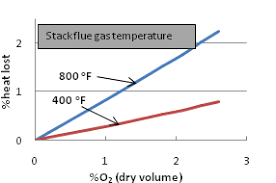The image above represents %heat loss in flue gas.

To compute for %heat loss in flue gas, four essential parameters are needed and these parameters are Mass (m), Specific Heat of Stock (Cp), Change in Temperature (ΔT) and Gross Calorific Value of Fuel (GCVf).

The formula for calculating %heat loss in fuel gas:

%QL = 100(mCpΔT/GCVf)

Where:

%QL = %Heat Loss in Flue Gas
m = Mass
Cp = Specific Heat of Stock
ΔT = Change in Temperature
GCVf = Gross Calorific Value of Fuel

Let’s solve an example;
Find the %heat loss in flue gas when the mass is 10, the specific heat of shock is 14, the change in temperature is 12 and the gross calorific value of fuel is 18.

This implies that;

m = Mass = 10
Cp = Specific Heat of Stock = 14
ΔT = Change in Temperature = 12
GCVf = Gross Calorific Value of Fuel = 18

%QL = 100(mCpΔT/GCVf)
%QL = 100(10(14)(12)/18)
%QL = 100(1680/18)
%QL = 100(93.33)
%QL = 9333.3

Therefore, the %heat loss of flue gas is 9333.3%.

## How to Calculate and Solve for Electron Kinetic Energy | X-Ray Crystallography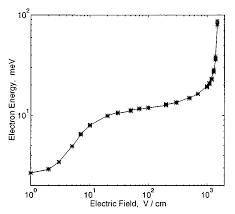The image above represents electron kinetic energy.

To compute for electron kinetic energy, two essential parameters are needed and these parameters are Mass (m) and Velocity (v).

The formula for calculating electron kinetic energy:

eV = mv²/2

Where:

eV = Electron Kinetic Energy
m = Mass
v = Velocity

Let’s solve an example;
Find the electron kinetic energy when the mass is 12 and velocity is 24.

This implies that;

m = Mass = 12
v = Velocity = 24

eV = mv²/2
eV = (12)(24)²/2
eV = (12)(576)/2
eV = (6912)/2
eV = 3456

Therefore, the electron kinetic energy is 3456 J.

Calculating the Mass when the Electron Kinetic Energy and the Velocity is Given.

m = eV x 2 / v2

Where;

m = Mass
eV = Electron Kinetic Energy
v = Velocity

Let’s solve an example;
Find the mass when the electron kinetic energy is 12 and the velocity is 4.

This implies that;

eV = Electron Kinetic Energy = 12
v = Velocity = 4

m = eV x 2 / v2
m = 12 x 2 / 42
m = 24 / 16
m = 1.5

Therefore, the mass is 1.5.

## How to Calculate and Solve for Velocity | De Broglie’s Law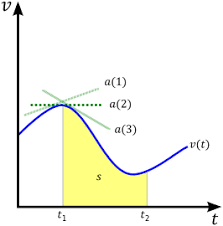The image above represents velocity.

To compute for velocity, three essential parameters are needed and these parameters are Planck’s Constant (h), Wavelength (λ) and Mass (m).

The formula for calculating velocity:

v = h / λm

Where;

v = velocity
λ = wavelength
h = Planck’s constant
m = mass

Let’s solve an example;
Find the velocity when the wavelength is 10, the planck’s constant is 6.626e-34 and the mass is 5.

This implies that;

λ = wavelength = 10
h = Planck’s constant = 6.626e-34
m = mass = 5

v = h / λm
v = 6.62607004e-34 (10)(5)
v = 6.62607004e-34 (50)
v = 1.33e-35

Therefore, the velocity is 1.33e-35 m/s.

## How to Calculate and Solve for Mass | De Broglie’s Law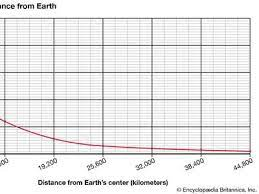The image above represents mass.

To compute for mass, three essential parameters are needed and these parameters are Planck’s Constant (h), Wavelength (λ) and Velocity (v).

The formula for calculating the mass:

m = h / λv

Where;

m = mass
λ = wavelength
h = Planck’s constant
v = velocity

Let’s solve an example;
Find the mass when the wavelength is 4, the planck’s constant is 6.626e-34 and the velocity is 2.

This implies that;

λ = wavelength = 4
h = Planck’s constant = 6.626e-34
v = velocity = 2

m = hλv
m = 6.62607004e-34(4)(2)
m = 6.62607004e-34(8)
m = 8.28e-35

Therefore, the mass is 8.28e-35 kg.

## How to Calculate and Solve for Wavelength | De Broglie’s Law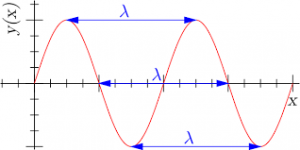The image above represents wavelength.

To compute for wavelength, three essential parameters are needed and these parameters are Planck’s Constant (h), Mass (m) and Velocity (v).

The formula for calculating wavelength:

λ = hmv

Where

λ = wavelength
h = Planck’s constant
m = mass
v = velocity

Let’s solve an example;
Find the wavelength when the planck’s constant is 6.62e-34, the mass is 21 and the velocity is 11.

This implies that;

h = Planck’s constant = 6.62e-34
m = mass = 21
v = velocity = 11

λ = hmv
λ = 6.62607004e-34(21)(11)
λ = 6.62607004e-34(231)
λ = 2.86e-36

Therefore, the wavelength is 2.86e-36 m.

Calculating the Mass when the Wavelength, the Planck’s Constant and the Velocity is Given.

m = h / λv

Where;

m = mass
λ = wavelength
h = Planck’s constant
v = velocity

Let’s solve an example;
Find the mass when the wavelength is 18, the planck’s constant is 6.626e-34 and the velocity is 6.

This implies that;

λ = wavelength = 18
h = Planck’s constant = 6.626e-34
v = velocity = 6

m = h / λv
m = 18 / 6.626e-34 x 6
m = 18 / 3.976e-33
m = 4.53

Therefore, the mass is 4.53 m.

## How to Calculate and Solve for Reaction: Lift Falls Freely | Motion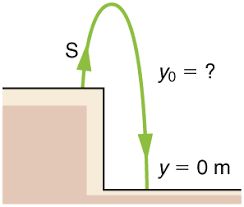The image above represents reaction: lift falls freely.

To compute for reaction: lift falls freely, two essential parameters are needed and these parameters are mass (m) and acceleration due to gravity (g).

The formula for calculating reaction: lift falls freely:

R = mg

Where;

R = Reaction
m = Mass
g = acceleration due to gravity

Let’s solve an example;
Find the reaction when the mass is 30 and acceleration due to gravity is 9.8.

This implies that;

m = Mass = 30
g = acceleration due to gravity = 9.8

R = mg
R = 30 x 9.8
R = 294

Therefore, the reaction is 294 N.

Calculating the Mass when the Reaction and the Acceleration due to Gravity is Given.

m = R / g

Where;

m = Mass
R = Reaction
g = acceleration due to gravity

Let’s solve an example;
Find the mass when the reaction is 40 and the acceleration due to gravity is 9.8

This implies that;

R = Reaction = 40
g = acceleration due to gravity = 9.8

m = R / g
m = 40 / 9.8
m = 40 / 9.8
m = 4.08

Therefore, the mass is 4.08.

## How to Calculate and Solve for Reaction: Lift Moves Down | Motion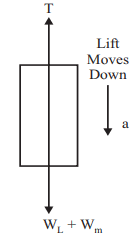The image above represents reaction: lift moves down.

To compute for reaction: lift moves down, three essential parameters are needed and these parameters are mass (m), acceleration (a) and acceleration due to gravity (g).

The formula for calculating reaction: lift moves down:

R = m(g – a)

Where;

R = Reaction
m = Mass
g = acceleration due to gravity
a = Acceleration

Let’s solve an example;
Find the reaction when the mass is 28, acceleration is 9 and acceleration due to gravity is 9.8.

This implies that;

m = Mass = 28
g = acceleration due to gravity = 9.8
a = Acceleration = 9

R = m(g – a)
R = 28(9.8 – 9)
R = 28(0.80)
R = 22.4

Therefore, the reaction is 22.4 N.

Calculating the Mass when the Reaction, the Acceleration and the Acceleration due to Gravity is Given.

m = R / g – a

Where;

m = Mass
R = Reaction
g = acceleration due to gravity
a = Acceleration

Let’s solve an example;
Find the mass when the reaction is 42, the acceleration is 8 and the acceleration due to gravity is 9.8

This implies that;

R = Reaction = 42
g = acceleration due to gravity = 9.8
a = Acceleration = 8

m = R / g – a
m = 42 / 9.8 + 8
m = 42 / 17.8
m = 2.359

Therefore, the mass is 2.359.

## How to Calculate and Solve for Reaction: Lift Moves Up | Motion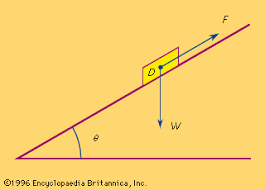The image above represents reaction: lift moves up.

To compute for reaction: lift moves up, three essential parameters are needed and these parameters are mass (m), acceleration (a) and acceleration due to gravity (g).

The formula for calculating reaction: lift moves up:

R = m(a + g)

Where;

R = Reaction
m = Mass
g = acceleration due to gravity
a = Acceleration

Let’s solve an example;
Find the reaction when the mass is 18, acceleration is 21 and acceleration due to gravity is 9.8.

This implies that;

m = Mass = 18
g = acceleration due to gravity = 21
a = Acceleration = 9.8

R = m(a + g)
R = 18(21 + 9.8)
R = 18(30.8)
R = 554.4

Therefore, the reaction is 554.4 N.

Calculating the Mass when the Reaction, the Acceleration and the Acceleration due to Gravity is Given.

m = R / a + g

Where;

m = Mass
R = Reaction
g = acceleration due to gravity
a = Acceleration

Let’s solve an example;
Find the mass when the reaction is 42, the acceleration is 21 and the acceleration due to gravity is 9.8

This implies that;

R = Reaction = 42
g = acceleration due to gravity = 9.8
a = Acceleration = 21

m = R / a + g
m = 42 / 21 + 9.8
m = 42 / 30.8
m = 1.36

Therefore, the mass is 1.36.

## How to Calculate and Solve for Force | Motion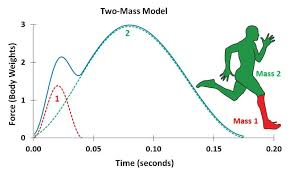The image above represents force.

To compute for force, two essential parameters are needed and these parameters are mass (m) and acceleration (a).

The formula for calculating force:

F = ma

Where;

F = Force
m = Mass
a = Acceleration

Let’s solve an example;
Find the force when the mass is 12 and the acceleration is 26?

This implies that;

m = Mass = 12
a = Acceleration = 26

F = ma
F = 12 x 26
F = 312

Therefore, the force is 312 N.

Calculating for Mass when the Force and the Acceleration is Given.

m = F / a

Where;

m = Mass
F = Force
a = Acceleration

Let’s solve an example;
Find the mass when the force is 100 and the acceleration is 20.

This implies that;

F = Force = 100
a = Acceleration = 20

m = F / a
m = 100 / 20
m = 5

Therefore, the mass is 5.

## How to Calculate and Solve for Linear Momentum | Motion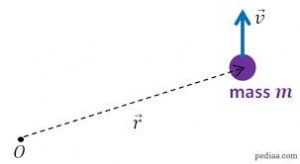The image above represents linear momentum.

To compute for linear momentum, two essential parameters are needed and these parameters are mass (m) and velocity (v).

The formula for calculating linear momentum:

p = mv

Where;

p = Momentum
m = Mass
v = Velocity

Let’s solve an example;
Find the momentum when the mass is 8 and the velocity is 12.

This implies that;

m = Mass = 8
v = Velocity = 12

p = mv
p = 8 x 12
p = 96

Therefore, the momentum is 96 Kgm/s.

Calculating the Mass when the Momentum and the Velocity is Given.

m = p / v

Where;

m = Mass
p = Momentum
v = Velocity

Let’s solve an example;
Find the mass when the momentum is 40 and the velocity is 18.

This implies that;

p = Momentum = 40
v = Velocity = 18

m = p / v
m = 40 / 18
m = 2.22

Therefore, the mass is 2.22 kg.